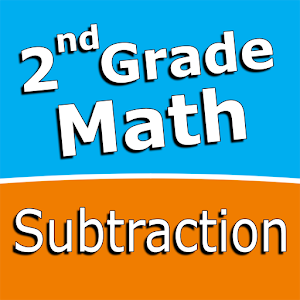Română

În fiecare zi oferim GRATUIT aplicații și jocuri Android licențiate pe care altfel ar trebui să le cumpărați.\$2.99
EXPIRAT

# Android Giveaway of the Day - Second grade Math - Subtraction

Intuitive interface powered by handwriting input and a fun and engaging mini game.
\$2.99 EXPIRAT
Părerea utilizatorilor: 1

This giveaway offer has been expired. Second grade Math - Subtraction is now available on the regular basis.

Intuitive interface powered by handwriting input and a fun and engaging mini game in addition to a regular math trainer mode make our app stand out from the crowd of generic math learning apps.

With Second grade Math - Subtraction you can practice and improve the following math skills:
- Subtraction facts - numbers up to 20
- Subtract a one-digit number from a two-digit number
- Subtract two two-digit numbers
- Subtract multiples of 10
- Subtract multiples of 100
- Subtract multiples of 10 or 100
- Subtract three-digit numbers
- Balance subtraction equations

Sergey Malugin

Education

### Versiune:

Varies with device

### Dimensiune:

Varies with device

Everyone

### Compatibilitate:

Varies with device

## Comentarii la Second grade Math - Subtraction

Thank you for voting!